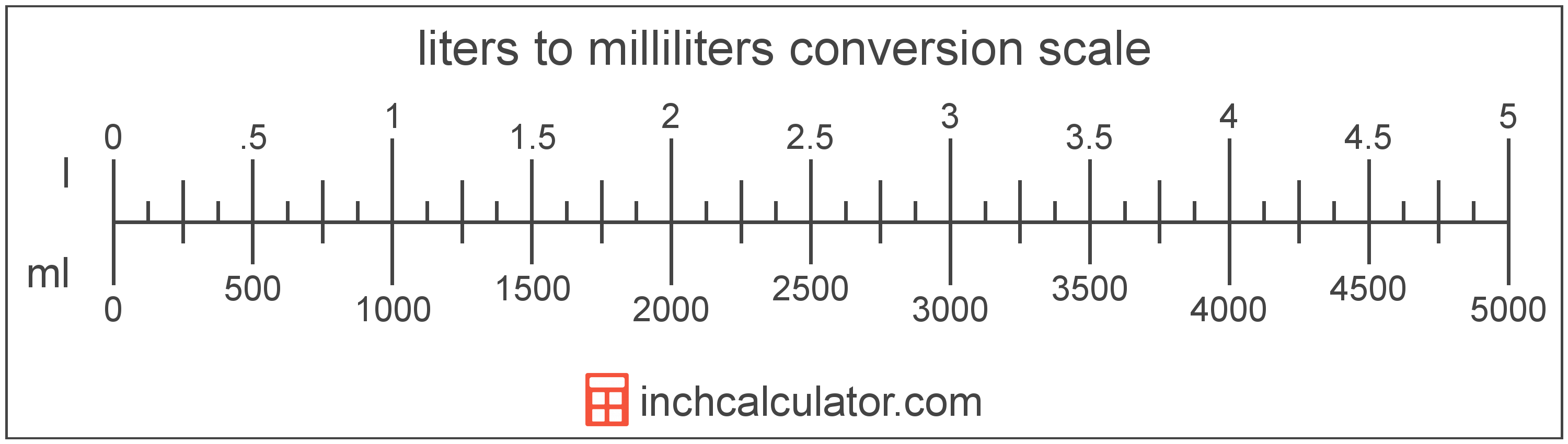# Milliliters to Liters Conversion

Enter the volume in milliliters below to get the value converted to liters.

Results in Liters:1 ml = 0.001 l

## How to Convert Milliliters to LitersTo convert a milliliter measurement to a liter measurement, divide the volume by the conversion ratio.

Since one liter is equal to 1,000 milliliters, you can use this simple formula to convert:

liters = milliliters ÷ 1,000

The volume in liters is equal to the milliliters divided by 1,000.

For example, here's how to convert 5,000 milliliters to liters using the formula above.
5,000 ml = (5,000 ÷ 1,000) = 5 l

## Milliliters

The milliliter is a unit of volume equal to 1 cubic centimeter, 1/1,000 of a liter, or about 0.061 cubic inches.

The milliliter is an SI unit of volume in the metric system. In the metric system, "milli" is the prefix for 10-3. A milliliter is sometimes also referred to as a millilitre. Milliliters can be abbreviated as ml, and are also sometimes abbreviated as mL or mℓ. For example, 1 milliliter can be written as 1 ml, 1 mL, or 1 mℓ.

Milliliters are sometimes expressed using the abbreviation cc for medical dosage, which is the abbreviation for the cubic centimeter.

## Liters

A liter is a unit of volume equal to 1,000 cubic centimeters. The liter is a special name defined for the cubic decimeter and is exactly equal to the volume of one cubic decimeter.

The liter is an SI accepted unit for volume for use with the metric system. A liter is sometimes also referred to as a litre. Liters can be abbreviated as l, and are also sometimes abbreviated as L or . For example, 1 liter can be written as 1 l, 1 L, or 1 ℓ.

## Milliliter to Liter Conversion Table

Milliliter measurements converted to liters
Milliliters Liters
1 ml 0.001 l
2 ml 0.002 l
3 ml 0.003 l
4 ml 0.004 l
5 ml 0.005 l
6 ml 0.006 l
7 ml 0.007 l
8 ml 0.008 l
9 ml 0.009 l
10 ml 0.01 l
20 ml 0.02 l
30 ml 0.03 l
40 ml 0.04 l
50 ml 0.05 l
60 ml 0.06 l
70 ml 0.07 l
80 ml 0.08 l
90 ml 0.09 l
100 ml 0.1 l
200 ml 0.2 l
300 ml 0.3 l
400 ml 0.4 l
500 ml 0.5 l
600 ml 0.6 l
700 ml 0.7 l
800 ml 0.8 l
900 ml 0.9 l
1,000 ml 1 l

## References

1. National Institute of Standards and Technology, Specifications, Tolerances, and Other Technical Requirements for Weighing and Measuring Devices, Handbook 44 - 2019 Edition, https://nvlpubs.nist.gov/nistpubs/hb/2019/NIST.HB.44-2019.pdf
2. National Institute of Standards and Technology, Units outside the SI, https://physics.nist.gov/cuu/Units/outside.html